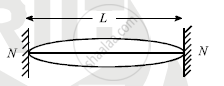HSC Science (General) 12th Board ExamMaharashtra State Board
Share

Books Shortlist

# Derive an Expression for Frequency in Fundamental Mode - HSC Science (General) 12th Board Exam - Physics

ConceptReflection of Transverse and Longitudinal Waves

#### Question

A wire of density ‘ρ’ and Young’s modulus ‘Y’ is stretched between two rigid supports separated by a distance ‘L’ under tension ‘T’. Derive an expression for its frequency in fundamental mode. Hence show that n=1/(2L)sqrt((Yl)/(rhoL)) where symbols have their usual meanings

#### Solution

onsider a string of lenght ‘L’. Let ‘m’ be the mass per unit length of the stirng ‘T’ be tension in the string.

If transveres wave is produced in the string, the velocity of the wave is given by v=sqrtT/m""....(1)If the string is plucked in middle, two incident and reflected wave will produce stationary wave. The string will vibrate in different mode which is called mode of vibrations.

The simplest mode of vibration is as shown in figure, which is called fundamental mode of vibration. Here two nodes and one antinode is formed. Let λ and n be corresponding wavelength and frequency

∴ L=λ/2    ∴λ=2L     ∴v=nλ=n2L

:."From equation (i)"n(2L)=sqrt(T/m)

:.n=1/(2L)sqrt(T/m)" ....(2)"

This is called fundamental frequency

Young’s modulus

Y=(TL)/(Al)

T=(YAl)/L, m=M/L=(Vrho)/L

=(ALrho)/L=Arho

:."from equation (2) "n=1/(2L)sqrt(((YAl)/L)/(Arho))

n=1/(2L)sqrt((Yl)/(AL))

Is there an error in this question or solution?

#### APPEARS IN

2013-2014 (March) (with solutions)
Question 3.2.1 | 5.00 marks

#### Video TutorialsVIEW ALL 

Solution Derive an Expression for Frequency in Fundamental Mode Concept: Reflection of Transverse and Longitudinal Waves.
S